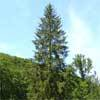#### You may also like### Two Trees

Two trees 20 metres and 30 metres long, lean across a passageway between two vertical walls. They cross at a point 8 metres above the ground. What is the distance between the foot of the trees?### Golden Triangle

Three triangles ABC, CBD and ABD (where D is a point on AC) are all isosceles. Find all the angles. Prove that the ratio of AB to BC is equal to the golden ratio.### Fitting In

The largest square which fits into a circle is ABCD and EFGH is a square with G and H on the line CD and E and F on the circumference of the circle. Show that AB = 5EF. Similarly the largest equilateral triangle which fits into a circle is LMN and PQR is an equilateral triangle with P and Q on the line LM and R on the circumference of the circle. Show that LM = 3PQ

##### Age 14 to 18Challenge Level
This was a tough question! Only one correct answer to this: Joshua, at Chatham Grammar School for Boys, sent us a great solution. It was as follows:

Our first step should be to generalise the dimensions shown, for the nth person in the stadium.  We shall let the point which must be visible to all be denoted as P.  The distance, d, from P to the nth person's seat is therefore given by:  $d_n = 10 + 0.8(n - 1) = 10 + 0.8n - 0.8 = 9.2 + 0.8n$. Furthermore, let $\theta_n$ represent the angle between the horizontal and the line of sight of the n-th person.  We assume every person has a torso height of 0.8m.  Let $x_n$ represent the height above ground level of the n-th person's seat.  We can consider the following:

$\theta_{n+1} = \arctan\left(\frac{1 + x_n}{d_n}\right)$.

This formula is much easier to consider when we break it down step by step.  Consider the zoomed in picture from the original problem.  Consider the right-angled triangle formed with the perpendicular at person 1's seat extended to the line of sight of person 2.  That triangle will enclose the angle between person 2's line of sight and the horizontal, $\theta_2$.  Its height is given by adding the overhead clearance (0.2m), the torso height (0.8m) and person 1's chair height ($x_1$).  Its base length is given by the distance from person 1's seat to the point P ($d_1$). This is true generally with 1 and 2 replaced by n and n+1, by considering similar diagrams.  Therefore by use of the expression $\tan\theta = \mathrm{opp}/\mathrm{adj}$, we arrive at the above general formula.

Now we can replace the $d_n$ in the formula with $9.2 + 0.8n$, to give:

$\theta_{n+1} = \arctan\left(\frac{1 + x_n}{9.2 + 0.8n}\right)$.

The last step now is to generate a recurrence relationship between $x_{n+1}$ and $x_n$, by eliminating $\theta$.  We can do this by getting an expression for $\theta_{n+1}$ in terms of $x_{n+1}$.  By again studying the zoomed in diagram, we shall get an expression for $x_2$ and then generalise.  Consider the triangle  formed between the perpendicular at person 2's seat up to their eye-level and the horizontal along to P.  This horizontal distance is $d_2$ and the vertical distance is $x_2 + 0.8$.  Therefore, by use of the tangent formula:

$\theta_{n+1} = \arctan\left(\frac{x_{n+1} + 0.8}{d_{n+1}}\right)$.

By eliminating $\theta_{n+1}$ from these two equations, we eventually end up with:

$x_{n+1} = \frac{2.64 + 0.16n + (10+0.8n)x_n}{9.2 + 0.8n}$

and we can now set up a table using this formula to calculate all of the values from $x_2$ to $x_{40}$, as we know that $x_1 = 0.4$.

The required values are:
$x_2 = 0.712$
$x_3 = 1.0388\dots$
$x_{40} = 19.0444\dots$

Fantastic!